Next: 4.4.3 Pattern Transfer Up: 4.4 Boolean Operations Previous: 4.4.1 Implementation

## 4.4.2 Chemical-Mechanical Planarization

If a geometry is represented as LS, Boolean operations can easily be calculated. They can be applied to simulate various semiconductor processes in a simplified manner. For example, the simplest model for chemical-mechanical planarization (CMP) assumes that everything is cut away above a certain height. Hence, the new structure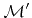is obtained by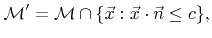(4.14)

where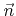is the normal vector and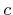the distance to the origin of the cutting plane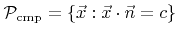. By setting up a LS function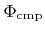, whose zero LS represents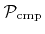(4.14) can be written as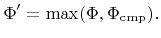(4.15)

Here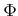andare the LS functions describing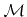and, respectively.

Figure 4.5 shows an example, for which CMP is used to flatten the geometry after an isotropic deposition process. Boolean operations are applied to both, the LS function representing the initial structure and the LS function representing the final structure after the deposition process.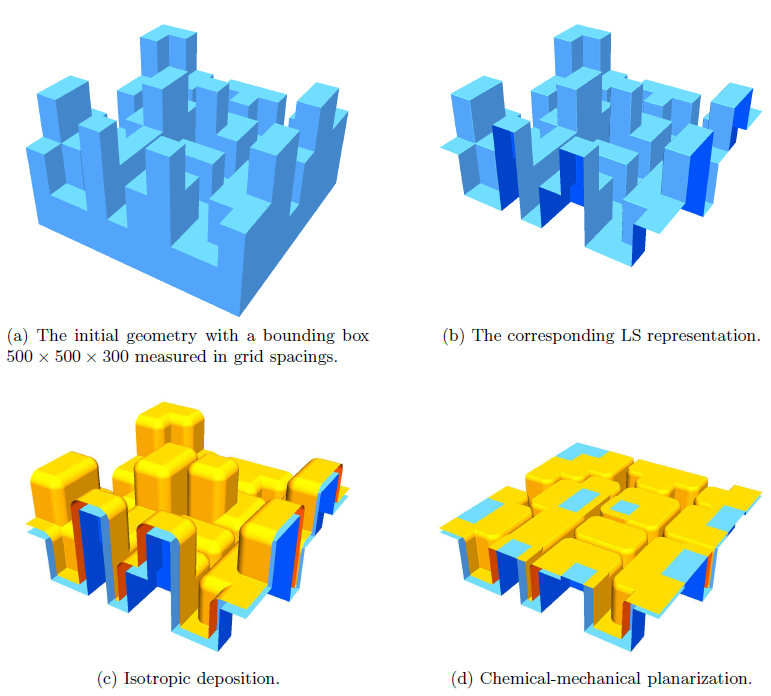Next: 4.4.3 Pattern Transfer Up: 4.4 Boolean Operations Previous: 4.4.1 Implementation

Otmar Ertl: Numerical Methods for Topography Simulation